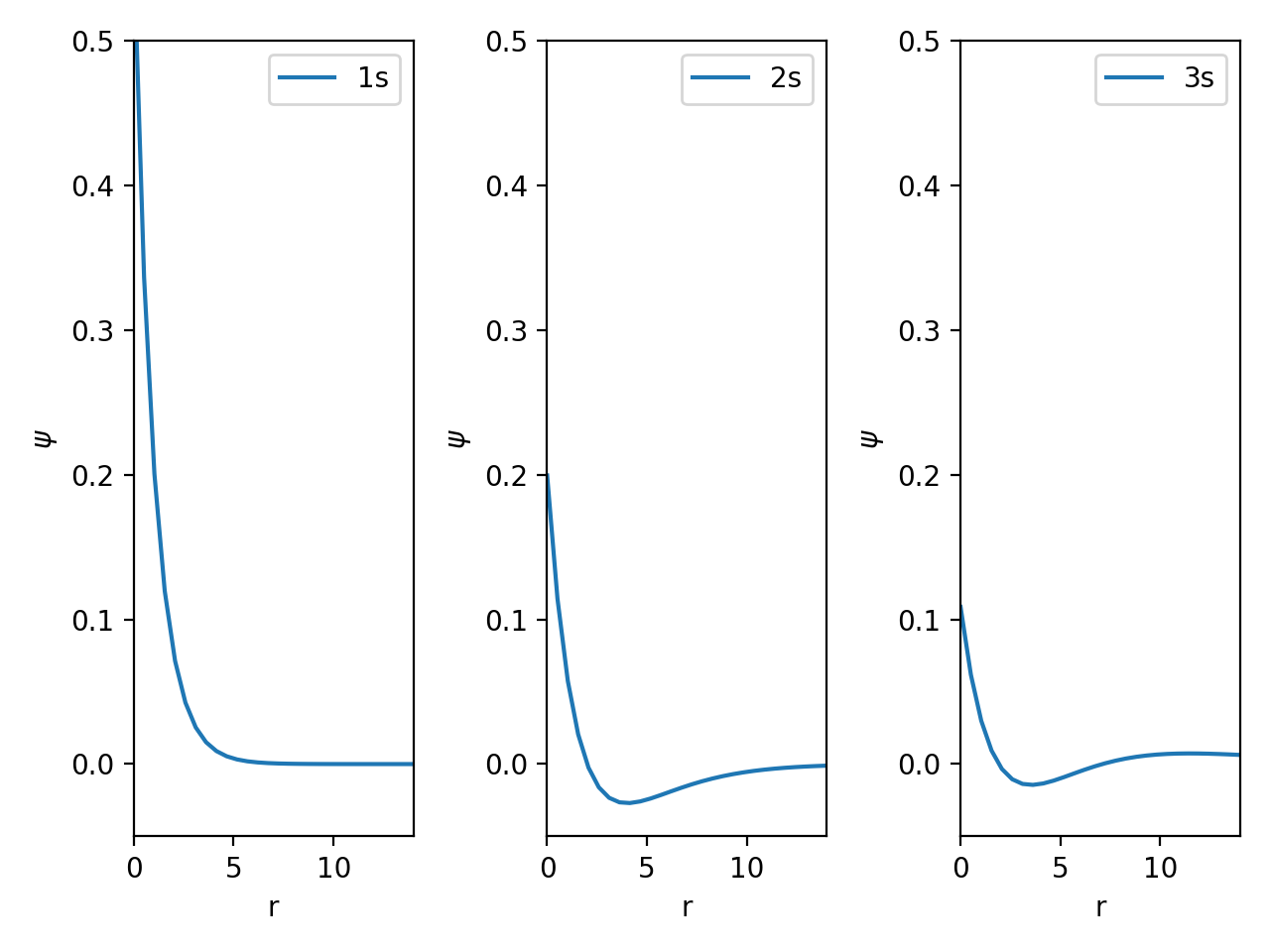# Subplots - Multiple Graphs on the same Figure#

Instead of displaying all three of our lines on the same plot, we might instead choose to display them side-by-side in different plots. We could use matplotlib to make three plots, then put them beside each other on our poster or in an image editing software. Fortunately, matplotlib will allow us to do this in our python program using subplots.

Subplots let you place several plots beside each other on a grid. We have already been using the plt.subplots command to create a single figure with one plot. To create a figure with multiple plots, we will put numbers inside the subplot command. These numbers will define the grid where we want to put figures. The first number will be how many rows we want on our plot, the second will be the number of columns.

Let’s try this a few times to see what happens.

import matplotlib.pyplot as plt

%matplotlib notebook

fig, ax = plt.subplots(2, 2)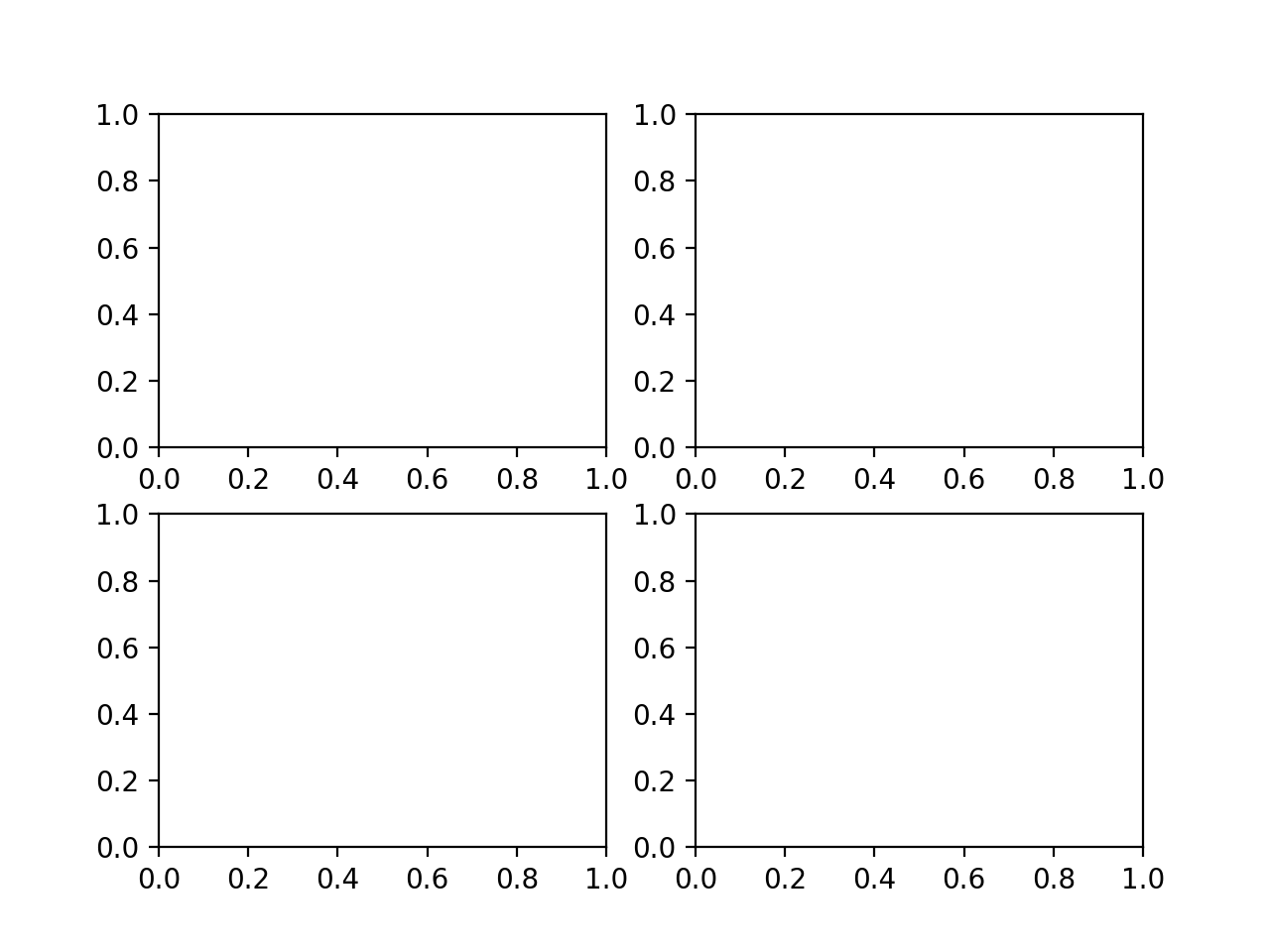## Using Subplots with our Data#

In the previous lesson, we plotted three data sets on the same graph. Instead of putting three data sets on the same graph, we might want to make three graphs side-by-side. We will use subplots for this.

First, we have to read in the data

import pandas as pd



Next, we create our figure and axes to work with. We want to make a graph with 1 row and 3 columns. The graphs axes labels appear to be overlapping when we do this, so we can use the fig.tight_layout command to improve spacing.

fig, ax = plt.subplots(1, 3)
fig.tight_layout()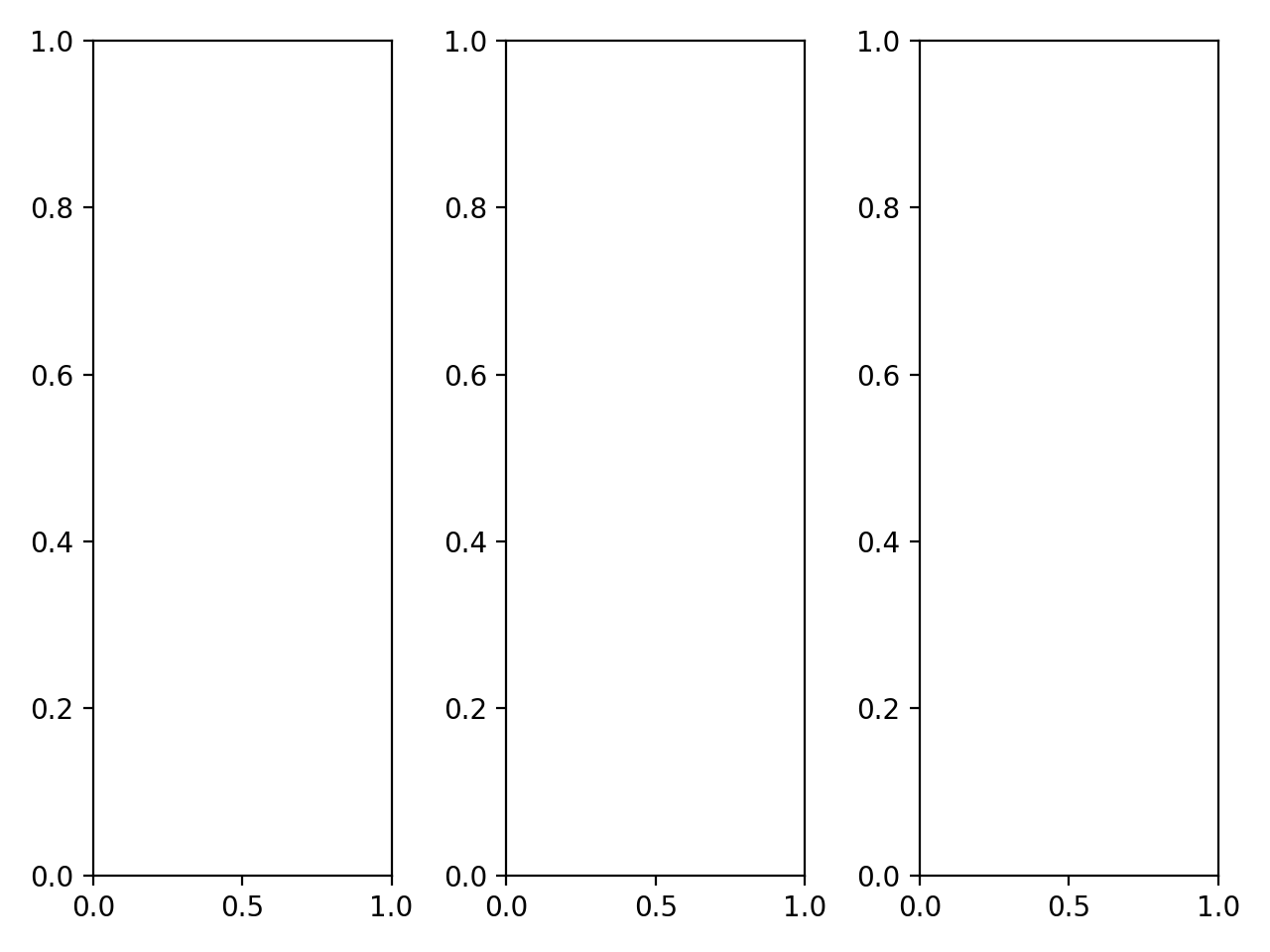The command above created a single figure which had plots on a grid. We told matplotlib that we wanted 1 row and 3 columns. Recall that in our previous lesson, ax was our figure axis that we added plots to. Now, ax is an array containing figure axes.

ax

array([<AxesSubplot:>, <AxesSubplot:>, <AxesSubplot:>], dtype=object)


Now, the ax variable is a list of figure axes. We can add plots to each of these in a way similar to what we used before. We just have to use slicing and indexing to get the axes we want to work with. For example, to access the first access we would use ax. You can see in the code block below that we have added a plot using this syntax.

fig, ax = plt.subplots(1, 3)
fig.tight_layout()
ax.plot("r","1s", data=s_orbitals)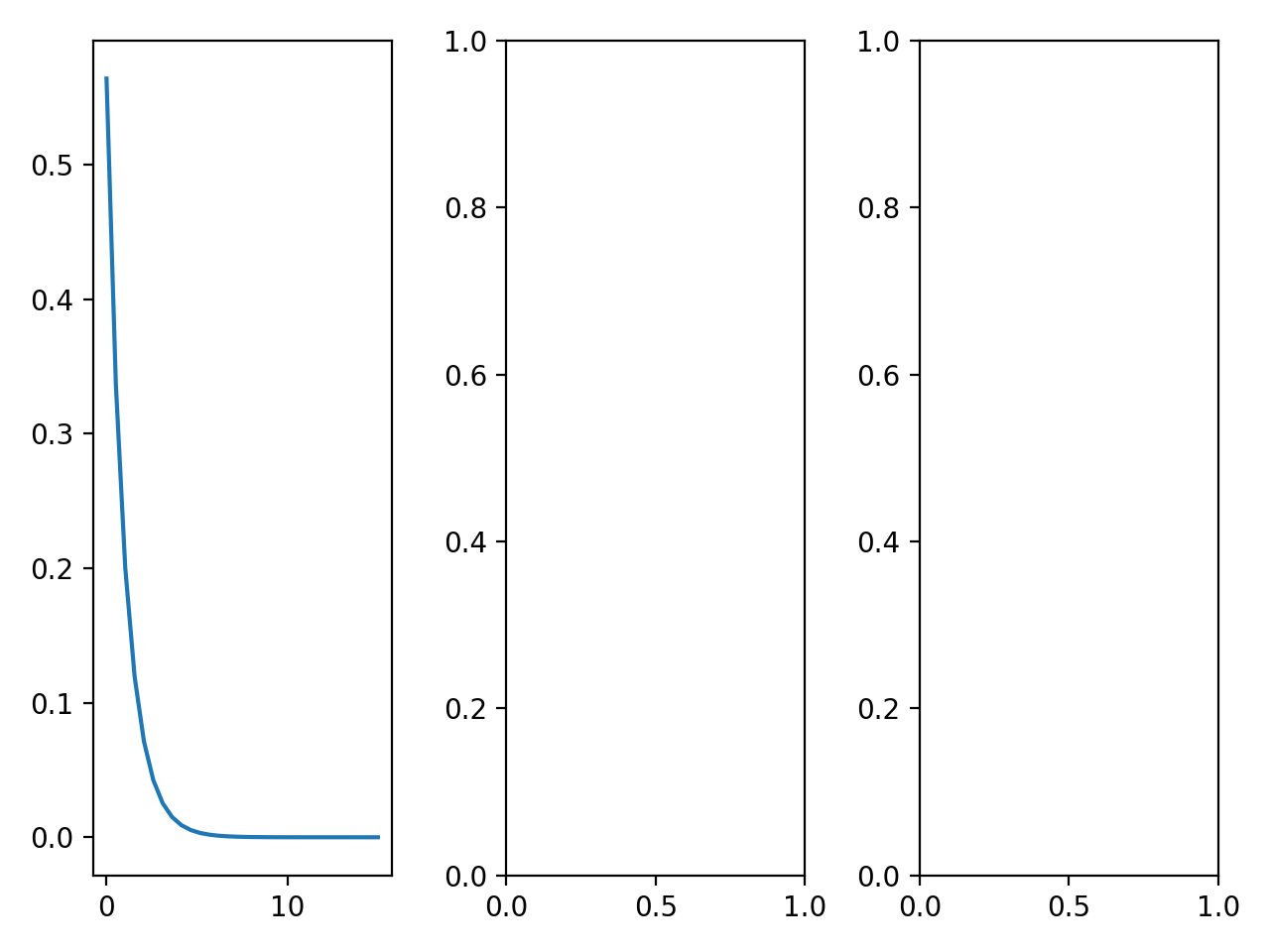[<matplotlib.lines.Line2D at 0x7fd599c4feb0>]


We can add data to the other axes.

fig, ax = plt.subplots(1, 3)

ax.plot("r","1s", data=s_orbitals)
ax.plot("r","2s", data=s_orbitals)
ax.plot("r","3s", data=s_orbitals)

fig.tight_layout()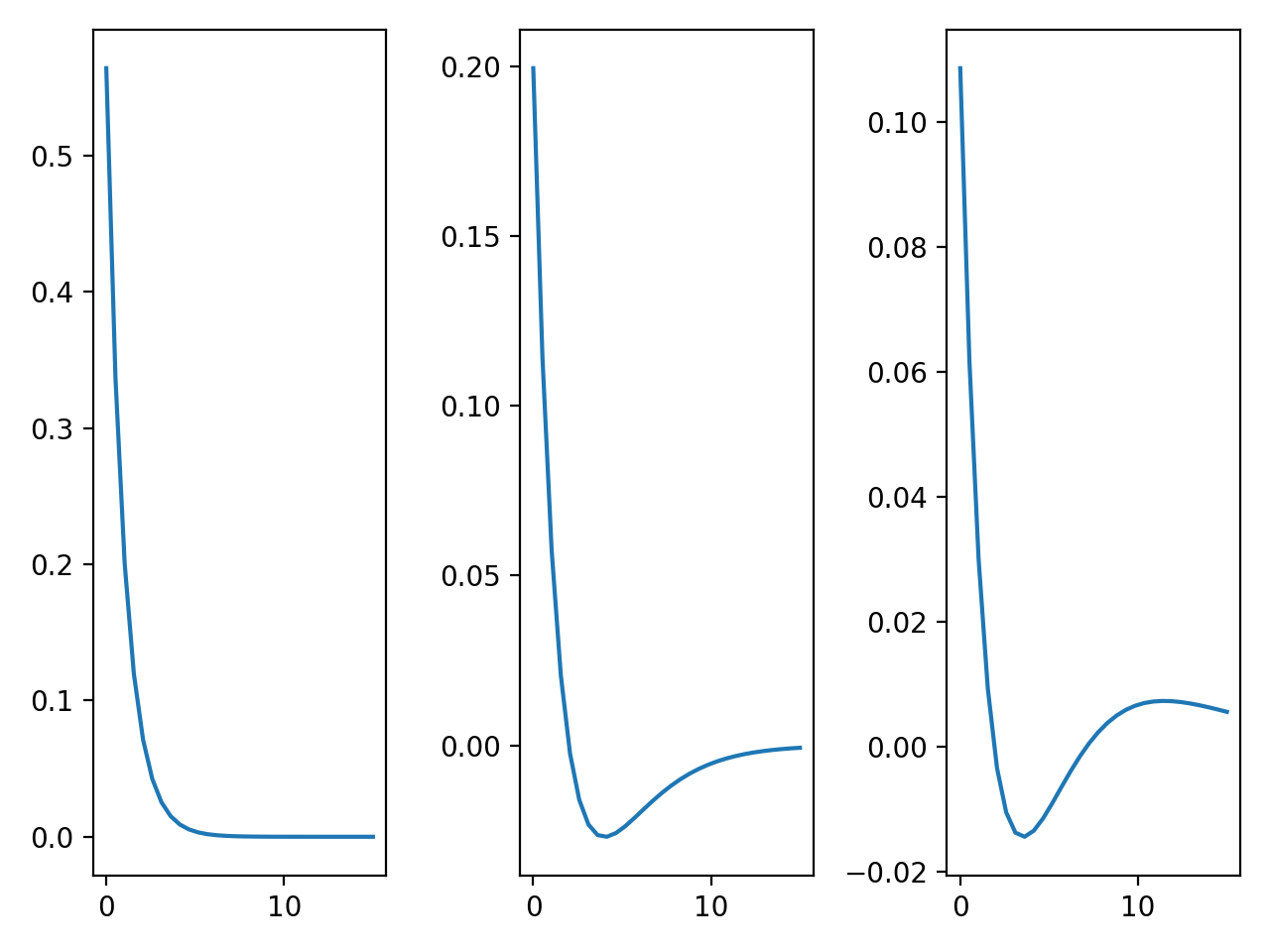All of the commands we learned previously can be used for subplots as well. The main difference is that you will slice into an array of axes, rather than applying it to the axes. We can add labels to our plots, for example.

Because there are so many axes, it starts to be conveneient to use a for loop to label the axes, especially if they should all have the same label.

fig, ax = plt.subplots(1, 3)

ax.plot("r","1s", data=s_orbitals)
ax.plot("r","2s", data=s_orbitals)
ax.plot("r","3s", data=s_orbitals)

for axis in ax:
axis.set_xlabel("r")
axis.set_ylabel(r"$\psi$")
axis.legend()

fig.tight_layout()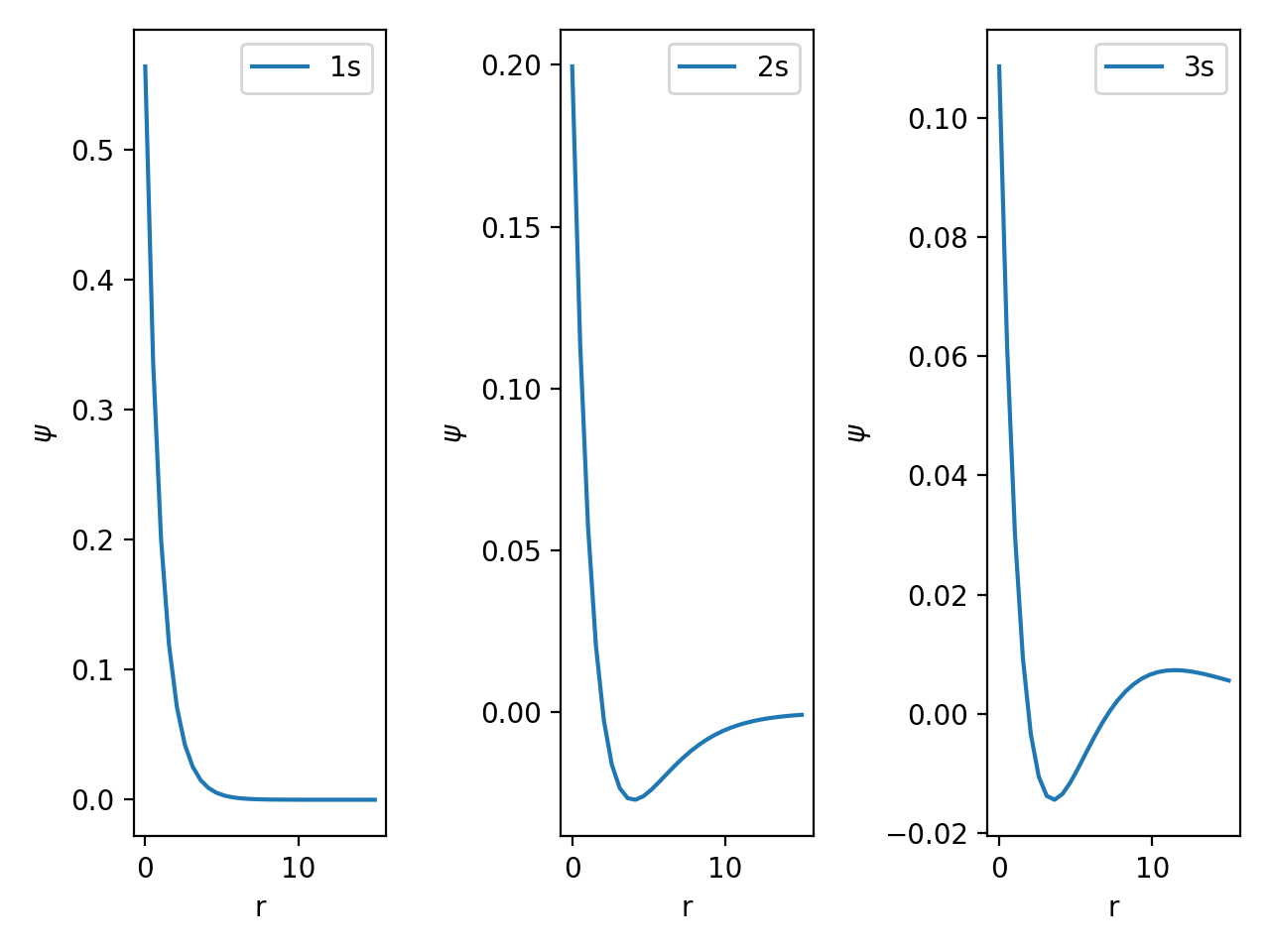## Considerations for Subplots#

If you are using subplots to display similar data, it is generally a good practice to use the same axis scales for all of the plots. You will notice that for the figure we created above, each y axis is on a different scale. It is much harder, and requires much more work from the plot reader to realize that the values for 3s are lower than those for 1s.

We can use the set_xlim and set_ylim commands to make sure that all of the plots are on the same scale.

fig, ax = plt.subplots(1, 3)

ax.plot("r","1s", data=s_orbitals)
ax.plot("r","2s", data=s_orbitals)
ax.plot("r","3s", data=s_orbitals)

for axis in ax:
axis.set_xlabel("r")
axis.set_ylabel(r"$\psi$")
axis.legend()
axis.set_xlim(0, 14)
axis.set_ylim(-0.05, 0.5)

fig.tight_layout()